4715.0 - National Aboriginal and Torres Strait Islander Health Survey, 2018-19
Latest ISSUE Released at 11:30 AM (CANBERRA TIME) 11/12/2019
 Page tools: .mffeedback,#pjs { display :none; } Enable Javascript to Print PagesPrint AllEmail NotificationRSSSearch this Product RELIABILITY OF ESTIMATES Two types of error are possible in estimates based on a sample survey: non-sampling error sampling error NON-SAMPLING ERROR Non-sampling error is caused by factors other than those related to sample selection. It is any factor that results in the data values not accurately reflecting the true value of the population. It can occur at any stage throughout the survey process. Examples include: selected persons that do not respond (e.g. refusals, non-contact) questions being misunderstood responses being incorrectly recorded errors in coding or processing the survey data. SAMPLING ERROR Sampling error is the expected difference that can occur between the published estimates and the value that would have been produced if the whole population had been surveyed. STANDARD ERROR One measure of sampling error is the standard error (SE). There are about two chances in three that an estimate will differ by less than one SE from the figure that would have been obtained if the whole population had been included. There are about 19 chances in 20 that an estimate will differ by less than two SEs. RELATIVE STANDARD ERROR The relative standard error (RSE) is a useful measure of sampling error. It is the SE expressed as a percentage of the estimate: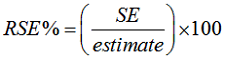RSEs for published estimates are supplied in Excel data tables, available via the Downloads tab. Only estimates with RSEs less than 25% are considered reliable for most purposes. Estimates with larger RSEs, between 25% and less than 50% have been included in the publication, but are flagged to indicate they are subject to high SEs. These should be used with caution. Estimates with RSEs of 50% or more have also been flagged and are considered unreliable for most purposes. STANDARD ERRORS OF PROPORTIONS AND PERCENTAGES A measure of sampling error can be calculated for proportions formed from the ratio of two estimates. For proportions where the denominator (y) is an estimate of the number of persons in a group and the numerator (x) is the number of persons in a sub-group of the denominator, the formula to approximate the RSE is given below. The formula is only valid when x is a subset of y: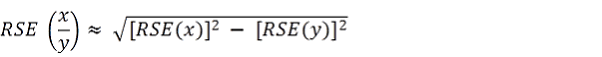COMPARISON OF ESTIMATES Published estimates can be used to calculate the difference between two survey estimates. The sampling error of the difference between two estimates depends on their SEs and the relationship (correlation) between them. An approximate SE of the difference between two estimates (x and y) may be calculated by the following formula: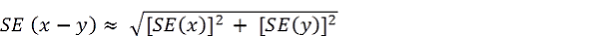While the above formula will only be exact for differences between unrelated characteristics of sub-populations, it is expected that it will provide a reasonable approximation for other data comparisons. MARGINS OF ERROR Another measure of sampling error is the Margin of Error (MOE). This describes the distance from the population value that the sample estimate is likely to be within. It is specified at a given level of confidence. Confidence levels typically used are 90%, 95% and 99%. For example, at the 95% confidence level, the MOE indicates that there are about 19 chances in 20 that the estimate will differ by less than the specified MOE from the population value (the figure obtained if the whole population had been enumerated). The 95% MOE is calculated as 1.96 multiplied by the SE: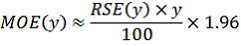The MOEs in this publication are calculated at the 95% confidence level. This can easily be converted to a 90% confidence level by multiplying the MOE by: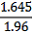or to a 99% confidence level by multiplying the MOE by: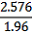CONFIDENCE INTERVALS A confidence interval expresses the sampling error as a range in which the population value is expected to lie at a given level of confidence. The confidence interval can easily be constructed from the MOE by taking the estimate plus or minus the MOE of the estimate. SIGNIFICANCE TESTING When comparing estimates between surveys or between populations within a survey, it is useful to determine whether apparent differences are 'real' differences or simply the product of differences between the survey samples. One way to examine this is to determine whether the difference between the estimates is statistically significant. This is done by calculating the standard error of the difference between two estimates (x and y) and using that to calculate the test statistic using the formula below: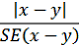where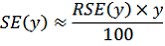If the value of the statistic is greater than 1.96, we can say there is good evidence of a statistically significant difference at 95% confidence levels between the two populations with respect to that characteristic. Otherwise, it cannot be stated with confidence that there is a real difference between the populations. Document Selection These documents will be presented in a new window.